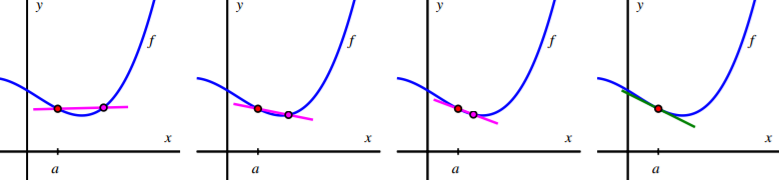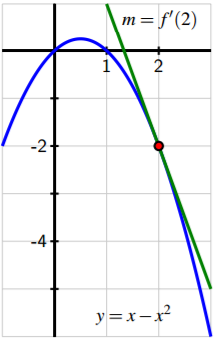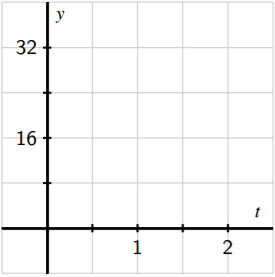$$\newcommand{\id}{\mathrm{id}}$$ $$\newcommand{\Span}{\mathrm{span}}$$ $$\newcommand{\kernel}{\mathrm{null}\,}$$ $$\newcommand{\range}{\mathrm{range}\,}$$ $$\newcommand{\RealPart}{\mathrm{Re}}$$ $$\newcommand{\ImaginaryPart}{\mathrm{Im}}$$ $$\newcommand{\Argument}{\mathrm{Arg}}$$ $$\newcommand{\norm}{\| #1 \|}$$ $$\newcommand{\inner}{\langle #1, #2 \rangle}$$ $$\newcommand{\Span}{\mathrm{span}}$$

2.7: Derivatives and Rates of Change

$$\newcommand{\vecs}{\overset { \rightharpoonup} {\mathbf{#1}} }$$

$$\newcommand{\vecd}{\overset{-\!-\!\rightharpoonup}{\vphantom{a}\smash {#1}}}$$

Skills to Develop

In this section, we strive to understand the ideas generated by the following important questions:

• How is the average rate of change of a function on a given interval defined, and what does this quantity measure?
• How is the instantaneous rate of change of a function at a particular point defined? How is the instantaneous rate of change linked to average rate of change?
• What is the derivative of a function at a given point? What does this derivative value measure? How do we interpret the derivative value graphically?
• How are limits used formally in the computation of derivatives?

Introduction

An idea that sits at the foundations of calculus is the instantaneous rate of change of a function. This rate of change is always considered with respect to change in the input variable, often at a particular fixed input value. This is a generalization of the notion of instantaneous velocity and essentially allows us to consider the question “how do we measure how fast a particular function is changing at a given point?” When the original function represents the position of a moving object, this instantaneous rate of change is precisely velocity, and might be measured in units such as feet per second. But in other contexts, instantaneous rate of change could measure the number of cells added to a bacteria culture per day, the number of additional gallons of gasoline consumed by going one mile per additional mile per hour in a car’s velocity, or the number of dollars added to a mortgage payment for each percentage increase in interest rate. Regardless of the presence of a physical or practical interpretation of a function, the instantaneous rate of change may also be interpreted geometrically in connection to the function’s graph, and this connection is also foundational to many of the main ideas in calculus.

In what follows, we will introduce terminology and notation that makes it easier to talk about the instantaneous rate of change of a function at a point. In addition, just as instantaneous velocity is defined in terms of average velocity, the more general instantaneous rate of change will be connected to the more general average rate of change. Recall that for a moving object with position function $$s$$, its average velocity on the time interval $$t=a$$ to $$t=a+h$$ is given by the quotient $AV_{[a,a+h]}=\frac{s(a+h)-s(a)}{h}.$

In a similar way, we make the following definition for an arbitrary function $$y=f(x)$$.

Definition 1.2

For a function $$f$$ , the average rate of change of $$f$$ on the interval $$[a, a+h]$$ is given by the value $AV_{[a,a+h]} =\frac{f(a+h) -f(a)}{h}.$

Equivalently, if we want to consider the average rate of change of $$f$$ on $$[a, b]$$, we compute $AV_{[a,b]}=\frac{f(b)-f(a)}{b-a}.$

It is essential to understand how the average rate of change of $$f$$ on an interval is connected to its graph.

Preview Activity $$\PageIndex{1}$$:

Suppose that $$f$$ is the function given by the graph below and that $$a$$ and $$a+h$$ are the input values as labeled on the x-axis. Use the graph in Figure $$\PageIndex{1}$$ to answer the following questions.Figure $$\PageIndex{1}$$: Plot of $$y=f(x)$$ for Preview Activity 1.3.

1. Locate and label the points $$(a, f(a))$$ and $$(a+h, f(a+h))$$ on the graph.
2. Construct a right triangle whose hypotenuse is the line segment from $$(a, f(a))$$ to $$(a+h, f(a+h))$$. What are the lengths of the respective legs of this triangle?
3. What is the slope of the line that connects the points $$(a, f(a))$$ and $$(a+h, f(a+h))$$?
4. Write a meaningful sentence that explains how the average rate of change of the function on a given interval and the slope of a related line are connected.

The Derivative of a Function at a Point

Just as we defined instantaneous velocity in terms of average velocity, we now define the instantaneous rate of change of a function at a point in terms of the average rate of change of the function $$f$$ over related intervals. In addition, we give a special name to “the instantaneous rate of change of $$f$$ at $$a$$,” calling this quantity “the derivative of $$f$$ at $$a$$,” with this value being represented by the shorthand notation $$f'(a)$$. Specifically, we make the following definition.

Definition 1.3

Let $$f$$ be a function and $$x=a$$ a value in the function’s domain. We define the derivative of $$f$$ with respect to $$x$$ evaluated at $$x=a$$, denoted $$f'(a)$$, by the formula $f'(a)=\lim_{h\to 0}\frac{f(a+h)-f(a)}{h},$

provided this limit exists. Aloud, we read the symbol $$f'(a)$$ as either “$$f$$ -prime at $$a$$” or “the derivative of $$f$$ evaluated at $$x=a$$.” Much of the next several chapters will be devoted to understanding, computing, applying, and interpreting derivatives. For now, we make the following important notes.

• The derivative of $$f$$ at the value $$x=a$$ is defined as the limit of the average rate of change of $$f$$ on the interval $$[a, a+h]$$ as $$h\to 0$$. It is possible for this limit not to exist, so not every function has a derivative at every point.
• We say that a function that has a derivative at $$x=a$$ is differentiable at $$x=a$$.
• The derivative is a generalization of the instantaneous velocity of a position function: when $$y=s(t)$$ is a position function of a moving body, $$s'(a)$$ tells us the instantaneous velocity of the body at time $$t=a$$.
• Because the units on $$\frac{f(a+h)-f(a)}{h}$$ are “units of $$f$$ per unit of $$x$$,” the derivative has these very same units. For instance, if $$s$$ measures position in feet and $$t$$ measures time in seconds, the units on $$s'(a)$$ are feet per second.
• Because the quantity $$\frac{f(a+h)-f(a)}{h}$$ represents the slope of the line through $$(a, f(a))$$ and $$(a+h, f(a+h))$$, when we compute the derivative we are taking the limit of a collection of slopes of lines, and thus the derivative itself represents the slope of a particularly important line.

While all of the above ideas are important and we will add depth and perspective to them through additional time and study, for now it is most essential to recognize how the derivative of a function at a given value represents the slope of a certain line. Thus, we expand upon the last bullet item above.

As we move from an average rate of change to an instantaneous one, we can think of one point as “sliding towards” another. In particular, provided the function has a derivative at $$(a, f(a))$$, the point $$(a+h, f(a+h))$$ will approach $$(a, f(a))$$ as $$h\to 0$$. Because this process of taking a limit is a dynamic one, it can be helpful to use computing technology to visualize what the limit is accomplishing. While there are many different options3 , one of the best is a java applet in which the user is able to control the point that is moving. See the examples referenced in the footnote here, or consider building your own, perhaps using the fantastic free program Geogebra4 .

In Figure $$\PageIndex{2}$$, we provide a sequence of figures with several different lines through the points $$(a, f(a))$$ and $$(a+h, f(a+h))$$ that are generated by different values of $$h$$. These lines (shown in the first three figures in magenta), are often called secant lines to the curve $$y=f(x)$$. A secant line to a curve is simply a line that passes through two points that lie on the curve. For each such line, the slope of the secant line is $$m=\frac{f(a+h)-f(a)}{h}$$, where the value of $$h$$ depends on the location of the point we choose. We can see in the diagram how, as $$h\to 0$$, the secant lines start to approach a single line that passes through the point $$(a, f(a))$$. In the situation where the limit of the slopes of the secant lines exists, we say that the resulting value is the slope of the tangent line to the curve. This tangent line (shown in the right-most figure in green) to the graph of $$y=f(x)$$ at the point $$(a, f(a))$$ is the line through $$(a, f(a))$$ whose slope is $$m=f'(a)$$.Figure $$\PageIndex{2}$$: A sequence of secant lines approaching the tangent line to $$f$$ at $$(a, f(a))$$.

As we will see in subsequent study, the existence of the tangent line at $$x=a$$ is connected to whether or not the function $$f$$ looks like a straight line when viewed up close at $$(a, f(a))$$, which can also be seen in Figure $$\PageIndex{3}$$, where we combine the four graphs in Figure $$\PageIndex{2}$$ into the single one on the left, and then we zoom in on the box centered at $$(a, f(a))$$, with that view expanded on the right (with two of the secant lines omitted). Note how the tangent line sits relative to the curve $$y=f(x)$$ at $$(a, f(a))$$ and how closely it resembles the curve near $$x=a$$.

3For a helpful collection of java applets, consider the work of David Austin of Grand Valley State University at http://gvsu.edu/s/5r, and the particularly relevant example at http://gvsu.edu/s/5s. For applets that have been built in Geogebra, a nice example is the work of Marc Renault of Shippensburg University at http://gvsu.edu/s/5p, with the example at http://gvsu.edu/s/5q being especially fitting for our work in this section. There are scores of other examples posted by other authors on the internet.Figure $$\PageIndex{3}$$: A sequence of secant lines approaching the tangent line to $$f$$ at $$(a, f(a))$$. At right, we zoom in on the point $$(a, f(a))$$. The slope of the tangent line (in green) to $$f$$ at $$(a, f(a))$$ is given by $$f'(a)$$.

At this time, it is most important to note that $$f'(a)$$, the instantaneous rate of change of $$f$$ with respect to $$x$$ at $$x=a$$, also measures the slope of the tangent line to the curve $$y=f(x)$$ at $$(a, f(a))$$. The following example demonstrates several key ideas involving the derivative of a function.

Example $$\PageIndex{1}$$:

Example 1.3. For the function given by $$f(x)=x-x^2$$, use the limit definition of the derivative to compute $$f'(2)$$. In addition, discuss the meaning of this value and draw a labeled graph that supports your explanation.

Solution. From the limit definition, we know that $f'(2)=\lim_{h\to 0}\frac{f(2+h)-f(2)}{h}.$

Now we use the rule for $$f$$ , and observe that $$f(2)=2-2^2=-2$$ and $$f(2+h)=(2+h)-(2+h)^2$$. Substituting these values into the limit definition, we have that $f'(2)=\lim_{h\to 0} \frac{(2+h)-(2+h)^2-(-2)}{h} .$

Observe that with $$h$$ in the denominator and our desire to let $$h\to 0$$, we have to wait to take the limit (that is, we wait to actually let $$h$$ approach 0). Thus, we do additionalFigure $$\PageIndex{4}$$: The tangent line to $$y=x-x^2$$ at the point $$(2, -2)$$.

algebra. Expanding and distributing in the numerator,

$f'(2)=\lim_{h\to 0}\frac{2+h-4-4h-h^2+2}{h} .$

Combining like terms, we have $f'(2)=\lim_{h\to 0}\frac{-3h-h^2}{h}.$

Next, we observe that there is a common factor of $$h$$ in both the numerator and denominator, which allows us to simplify and find that $f'(2)=\lim_{h\to 0} (-3-h).$

Finally, we are able to take the limit as $$h\to 0$$, and thus conclude that $$f'(2)=-3$$.

Now, we know that $$f'(2)$$ represents the slope of the tangent line to the curve $$y=x-x^2$$ at the point $$(2, -2)$$; $$f'(2)$$ is also the instantaneous rate of change of $$f$$ at the point $$(2, -2)$$. Graphing both the function and the line through $$(2, -2)$$ with slope $$m=f'(2)=-3$$, we indeed see that by calculating the derivative, we have found the slope of the tangent line at this point, as shown in Figure 1.3.

The following activities will help you explore a variety of key ideas related to derivatives.

Activity $$\PageIndex{2}$$:

Consider the function $$f$$ whose formula is $$f(x)=3-2x$$.

1. What familiar type of function is $$f$$? What can you say about the slope of $$f$$ at every value of $$x$$?
2. Compute the average rate of change of $$f$$ on the intervals $$[1, 4], [3, 7]$$, and $$[5, 5+h]$$; simplify each result as much as possible. What do you notice about these quantities?
3. Use the limit definition of the derivative to compute the exact instantaneous rate of change of $$f$$ with respect to $$x$$ at the value $$a=1$$. That is, compute $$f'(1)$$ using the limit definition. Show your work. Is your result surprising?
4. Without doing any additional computations, what are the values of $$f'(2), f'(\pi)$$, and $$f'(-\sqrt{2})$$? Why?

Activity $$\PageIndex{3}$$:

A water balloon is tossed vertically in the air from a window. The balloon’s height in feet at time $$t$$ in seconds after being launched is given by $$s(t)=-16t^2+16t+32$$. Use this function to respond to each of the following questions.

1. Sketch an accurate, labeled graph of $$s$$ on the axes provided in Figure $$\PageIndex{5}$$. You should be able to do this without using computing technology.Figure $$\PageIndex{5}$$: Axes for plotting $$y=s(t)$$ in Activity 1.8

1. Compute the average rate of change of $$s$$ on the time interval $$[1, 2]$$. Include units on your answer and write one sentence to explain the meaning of the value you found.
2. Use the limit definition to compute the instantaneous rate of change of $$s$$ with respect to time, $$t$$, at the instant $$a=1$$. Show your work using proper notation, include units on your answer, and write one sentence to explain the meaning of the value you found.
3. On your graph in (a), sketch two lines: one whose slope represents the average rate of change of $$s$$ on $$[1, 2]$$, the other whose slope represents the instantaneous rate of change of $$s$$ at the instant $$a=1$$. Label each line clearly.
4. For what values of a do you expect $$s'(a)$$ to be positive? Why? Answer the same questions when “positive” is replaced by “negative” and “zero.”

Activity $$\PageIndex{4}$$:

A rapidly growing city in Arizona has its population $$P$$ at time $$t$$, where $$t$$ is the number of decades after the year 2010, modeled by the formula $$P(t)=25000e^{t/5}$$. Use this function to respond to the following questions.

1. Sketch an accurate graph of $$P$$ for $$t=0$$ to $$t=5$$ on the axes provided in Figure $$\PageIndex{6}$$. Label the scale on the axes carefully.Figure $$\PageIndex{6}$$: Axes for plotting $$y=P(t)$$ in Activity 1.9.

1. Compute the average rate of change of $$P$$ between 2030 and 2050. Include units on your answer and write one sentence to explain the meaning (in everyday language) of the value you found.
2. Use the limit definition to write an expression for the instantaneous rate of change of $$P$$ with respect to time, $$t$$, at the instant $$a=2$$. Explain why this limit is difficult to evaluate exactly.
3. Estimate the limit in (c) for the instantaneous rate of change of $$P$$ at the instant $$a=2$$ by using several small $$h$$ values. Once you have determined an accurate 30 estimate of $$P'(2)$$, include units on your answer, and write one sentence (using everyday language) to explain the meaning of the value you found.
4. On your graph above, sketch two lines: one whose slope represents the average rate of change of $$P$$ on $$[2, 4]$$, the other whose slope represents the instantaneous rate of change of $$P$$ at the instant $$a=2$$.
5. In a carefully-worded sentence, describe the behavior of $$P'(a)$$ as a increases in value. What does this reflect about the behavior of the given function $$P$$?

Summary

In this section, we encountered the following important ideas:

• The average rate of change of a function $$f$$ on the interval $$[a, b]$$ is $$\frac{f(b)-f(a)}{b-a}$$. The units on the average rate of change are units of $$f$$ per unit of $$x$$, and the numerical value of the average rate of change represents the slope of the secant line between the points $$(a, f(a))$$ and $$(b, f(b))$$ on the graph of $$y=f(x)$$. If we view the interval as being $$[a, a+h]$$ instead of [a, b], the meaning is still the same, but the average rate of change is now computed by $$\frac{f(a+h)-f(a)}{h}$$ .
• The instantaneous rate of change with respect to $$x$$ of a function $$f$$ at a value $$x=a$$ is denoted $$f'(a)$$ (read “the derivative of $$f$$ evaluated at $$a$$” or “$$f$$ -prime at $$a$$”) and is defined by the formula $f'(a)=\lim_{h\to 0}\frac{f(a+h)-f(a)}{h} ,$

provided the limit exists. Note particularly that the instantaneous rate of change at $$x=a$$ is the limit of the average rate of change on $$[a, a+h]$$ as $$h\to 0$$.

• Provided the derivative $$f'(a)$$ exists, its value tells us the instantaneous rate of change of $$f$$ with respect to $$x$$ at $$x=a$$, which geometrically is the slope of the tangent line to the curve $$y=f(x)$$ at the point $$(a, f(a))$$. We even say that $$f'(a)$$ is the slope of the curve $$y=f(x)$$ at the point $$(a, f(a))$$.
• Limits are the link between average rate of change and instantaneous rate of change: they allow us to move from the rate of change over an interval to the rate of change at a single point.

Contributors

Matt Boelkins (Grand Valley State University), David Austin (Grand Valley State University), Steve Schlicker (Grand Valley State University)